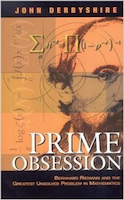## The Zeta songThis piece of mathematical doggerel was written by Tom Apostol (lines 1-32, 1955) and Saunders MacLane (lines 33-40, 1973); it was included as an appendix in John Derbyshire's book about the Riemann Hypothesis, Prime Obsession (2003). This translation accompanied the Russian edition (Prostaya Oderzhimost', A.M. Semikhatov, 2008).

zeta.pdf (depending on how your .pdf reader is set up, you might want to zoom well in, or else some m-dashes might be invisible)

Some notes for non-mathematicians:

• A "zero" of a function is a value of the variable for which the function equals zero.
• "Complex numbers" are numbers of the form a + bi, where a and b are real numbers and i is the square root of -1; "zeta of s" is a certain complex-valued function of a complex variable (traditionally written as s = sigma + it). The Riemann Hypothesis -- currently, the best-known open problem in mathematics -- states that all "non-trivial" zeros of zeta have real part 0.5. The line sigma = 0.5 is referred to as the "critical line," while the region 0 < sigma < 1 is known as the "critical strip."
• The letter "pi" is used twice, with different meanings. On line 4, it's the familiar constant 3.1415926... (the ratio of the circumference of a circle to its diameter); on line 17, it stands for the function pi(x), defined as the number of prime numbers smaller than x. The term "mod" is also used twice in the original, likewise with different meanings; in the Russian version, I tossed the first one out, since it wasn't really necessary.
• The so-called "natural" logarithm (having base e = 2.7182818...) is usually written as "log" in English, but as "ln" (pronounced "el'-EN") in Russian.
• The function Li(x) is the integral from 0 to x of (1 / ln t) dt. It is pronounced "el-EYE" in English, and just "li" in Russian.
• "TRPCh" = "teorema o raspredelenii prostykh chisel" (in English, "the prime number theorem") states that pi(x) is approximately equal to x / ln x. This approximation is what can be improved (or "strengthened"), typically using a technique called "contour integration."
• A field ("polye") is a certain type of algebraic object. Its "characteristic" can be either 0, or a prime number p. Statements similar to the R.H. have been proven for fields of prime characteristic; but the original conjecture concerns the field of complex numbers, which has characteristic 0.

BACK to "translations"